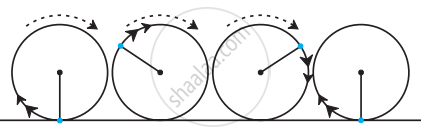# The radius of the wheel of a bus is 0.7 m. How many rotations will a wheel complete while travelling a distance of 22 km? - Mathematics

Sum

The radius of the wheel of a bus is 0.7 m. How many rotations will a wheel complete while traveling a distance of 22 km?#### Solution

Circumference of circle = πd = 22/7 × 1.4 = 4.4 m

When the wheel completes one rotation it crosses a distance of 4.4 m., (1 rotation = 1 circumference)

Total number of rotations = "distance"/"circumference"

= (22000)/(4.4)

= (220000)/(44)

= 5000

A wheel completes 10,000 rotations to cover a distance of 22 km.

Concept: Circumference of a Circle
Is there an error in this question or solution?